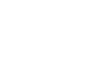What are Newtown law? - letsdiskussYash Surve

Student | Posted on | others

What are Newtown law?

0
0

| Posted on

Newton's law of motion describes the relationship between the motion of an object and the force acting on it.

Isaac Newton stated the three law of motion -

First law of motion - It is also known as law of inertia. A body at rest will remain at rest and a body at motion will remain in motion unless it is acted upon by an external force.

Second law of motion - The force acting on an object is equal to the mass of that object times it's acceleration.

Third law of motion- For every action there is an equal and opposite reaction.0
0